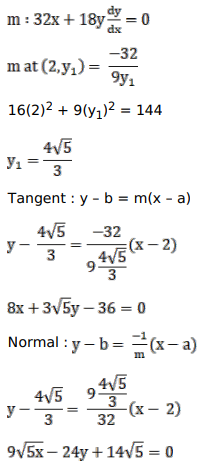# Find the equations of the tangent and the normal to the given curve at the indicated point for

Question:

Find the equations of the tangent and the normal to the given curve at the indicated point for

$16 \mathrm{x}^{2}+9 \mathrm{y}^{2}=144$ at $\left(2, \mathrm{y}_{1}\right)$, where $\mathrm{y}_{1}>0$

Solution: